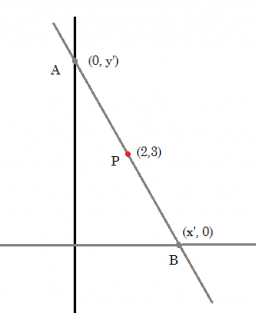# Intersections 49433

Draw a graph of the function given by the equation y = -2x +3, determine its intersections with the coordinate axes, and complete the missing coordinates A [3 ;? ], B [? ; 8].

x0 =  0
y0 =  3
x1 =  1.5
y1 =  0
Ay =  -3
Bx =  -2.5

### Step-by-step explanation:Did you find an error or inaccuracy? Feel free to write us. Thank you!